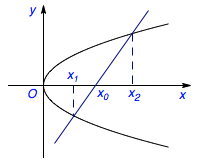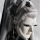# 抛物线的一则常用性质• 2014年北京丰台区高三期末考试

已知抛物线$C:y^2=2px(p>0)$的焦点为$F(1,0)$，点$O$为坐标原点，$A$，$B$是曲线$C$上异于$O$的两点．
（1）求曲线$C$的方程；
（2）若直线$OA$，$OB$的斜率之积为$-\frac 12$，求证：直线$AB$过定点．

• 2013年北京海淀区高三期末考试

已知$E(2,2)$是抛物线$C:y^2=2px$上一点，经过点$2,0$的直线$l$与抛物线$C$交于$A$，$B$两点（不同于点$E$），直线$EA$，$EB$分别交直线$x=-2$于点$M$，$N$．
（1）求抛物线方程及其焦点坐标；
（2）已知$O$为原点，求证：$\angle MON$为定值．

• 2013年北京西城区高三期末考试

已知抛物线$y^2=4x$的焦点为$F$，过点$P(2,0)$的直线交抛物线于$A(x_1,y_1)$，$B(x_2,y_2)$两点，直线$AF$，$BF$分别与抛物线交于点$M$，$N$．
（1）求$y_1y_2$的值；
（2）记直线$MN$的斜率为$k_1$，直线$AB$的斜率为$k_2$，证明：$\dfrac {k_1}{k_2}$为定值．

### 6 Responses to 抛物线的一则常用性质

1.Ivan说：

没有答案吗

•意琦行说：

没有，相信自己：）

2. Pingback引用通告： 2014年高考山东卷理科压轴题解答 | Math173

3. Pingback引用通告： 每日一题任他东南西北风（抛物线） | 数海拾贝内容系统

4. Pingback引用通告： 每日一题任他东南西北风 | Math173

5.临風往事说：

与直线x=my+x0,联立即可证明，不过您是怎么发现的呢